Next: Poisson's Equation Up: Electrostatic Fields Previous: Introduction

# Laplace's Equation

Laplace's equation is written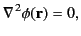(127)

where the functionis often referred to as a potential. Suppose that we wish to find a solution to this equation in some finite volume, bounded by a closed surface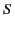, subject to the boundary condition(128)

whenlies on. Consider the vector identity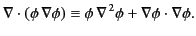(129)

Integrating this expression over, making use of the divergence theorem, we obtain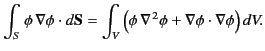(130)

It follows from Equations (127) and (128) that(131)

which implies thatthroughoutand on. Hence, Equation (128) yields(132)

throughoutand on. We conclude that the only solution to Laplace's equation, (127), subject to the boundary condition (128), is the trivial solution (132). Finally, if we let the surfacetend to infinity then we deduce that the only solution to Laplace's equation, (127), subject to the boundary conditionas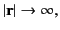(133)

is(134)

for all.

Consider a potentialthat satisfies Laplace's equation, (127), in some finite volume, bounded by the closed surface, subject to the boundary condition(135)

whenlies on. Here,is a known surface distribution. We can demonstrate that this potential is unique. Let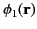and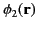be two supposedly different potentials that both satisfy Laplace's equation throughout, as well as the previous boundary condition on. Let us form the difference. This function satisfies Laplace's equation throughout, subject to the boundary condition(136)

whenlies on. However, as we have already seen, this implies that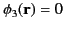throughoutand on. Hence,andare identical, and the potentialis therefore unique.Next: Poisson's Equation Up: Electrostatic Fields Previous: Introduction
Richard Fitzpatrick 2014-06-27